## Quiz 16 : Additional Topics in International Capital BudgetingLooking for Finance Homework Help?# Quiz 16 : Additional Topics in International Capital Budgeting

Weighted Average Cost of Capital: WACC is the weighted cost of capital and it is the blended cost of raising capital considering all sources of finance; equity and debt. The weight of debt is 0.4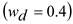The weight of equity is 0.6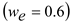The cost of debt is 8%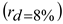The cost of equity is 15%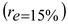The corporate tax is 34%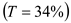The firm's WACC can be calculated using the formula given below: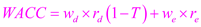Where,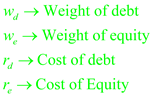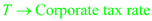Substitute the values in the formula: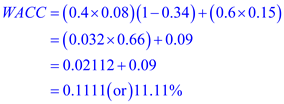So, the firm's WACC is 11.11 %

Explaining the reason for the required rate of return for a capital budgeting problem is project specific: In the context of project, the required rate of return is the minimum rate of return required by an investor to invest in a project, given the project's riskiness. The appropriate required rate of return for a project should be the project specific required rate of return. Required rate of return of a firm reflects risk of an average projects undertaken by the firm. If a new project is similar to the existing projects of firm, then the firm's required rate of return on capital invested can be used as that of project. But if the firm is undertaking a significantly different project compared to the existing projects that firm has already undertaken, then the firm's required rate of return does not appropriately cover the new project's cost of capital. If new project is riskier than the firm's existing projects, then it should charge a higher cost of capital and thereby it requires higher rate of return. Similarly, if new project is less risky than the firm's existing projects, then it should charge a lower cost of capital and thereby it requires lower rate of return.

Annual income from the project if the NPV is zero: First, calculate WACC with the provided values. The weight of debt is 0.35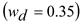, The weight of equity is 0.65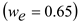The cost of debt is 9%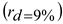The cost of equity is 22%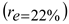The corporate tax rate is 40%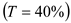The project's WACC can be calculated using the formula given below:Where,Substitute the values in the formula: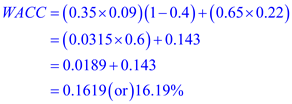The weighted average cost of capital is 16.19% The investment is 30 million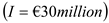The WACC is 16.19%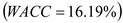The Net present value of the investment is 0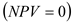The annual income from project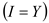can be calculated using the formula given below: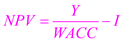Where,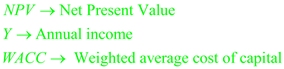Substitute the values in the formula: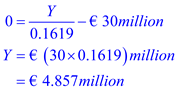So, the annual income from the project if it is to be a zero net present value investment must be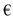4.857 million